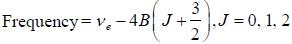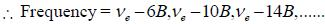Courses

# Test: Atomic & Molecular Physics - 2

## 20 Questions MCQ Test GATE Physics Mock Test Series | Test: Atomic & Molecular Physics - 2

Description
This mock test of Test: Atomic & Molecular Physics - 2 for GATE helps you for every GATE entrance exam. This contains 20 Multiple Choice Questions for GATE Test: Atomic & Molecular Physics - 2 (mcq) to study with solutions a complete question bank. The solved questions answers in this Test: Atomic & Molecular Physics - 2 quiz give you a good mix of easy questions and tough questions. GATE students definitely take this Test: Atomic & Molecular Physics - 2 exercise for a better result in the exam. You can find other Test: Atomic & Molecular Physics - 2 extra questions, long questions & short questions for GATE on EduRev as well by searching above.
*Answer can only contain numeric values
QUESTION: 1

### The rotational lines seperation in Raman experiment for HC1 molecule is 41.6 cm-1 The inter nuclear distance is________Å

Solution:

Rotational Raman line seperation is 4B = 41.6 cm-1
∴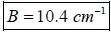Also,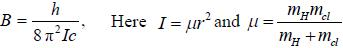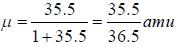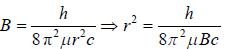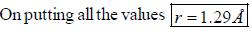*Answer can only contain numeric values
QUESTION: 2

### The rotational inertia for HI molecule is 4.5 x 10-48 kg m2. The temperature at which the average translational kinetic energy of molecule equals to difference of ground rotational static and First excited state is___________K.

Solution: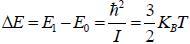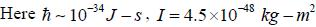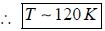*Answer can only contain numeric values
QUESTION: 3

### Total number of vibrational degree of freedom of H2 O2 is__________________

Solution:

Vibrational degree of freedom is 3N – 6 = 3 x 4 - 6 = 6

*Answer can only contain numeric values
QUESTION: 4

The number of Zeeman lines of the spectrum line 3D→ 3P1  in presence of weak magnetic field is______________

Solution: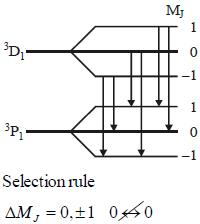*Answer can only contain numeric values
QUESTION: 5

For an atom in the state 2D5/2 the lande-g-factor should be__________

Solution: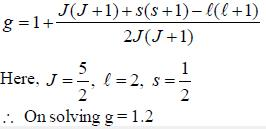*Answer can only contain numeric values
QUESTION: 6

Let E1, E2, E3, be the first three energy levels of H-atom. Consider the ratio (E3—E1)/(E2-E1). Neglecting the fine structure condition, this ratio is approximately____________

Solution: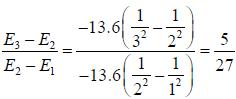*Answer can only contain numeric values
QUESTION: 7

Two monochromatic sources, L1 and L2 emit light at 600 nm and 700 nm respectively. If their frequency band width are 10-1 and 10-3 GHz respectively, then ratio of line width L1 and Lis approximately___________

Solution: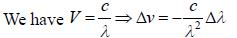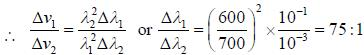QUESTION: 8

The figure (shown) depicts the energy levels of a 4 level atomic system with the Einstein A coefficients as follows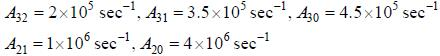The life time of the atomic level 3 is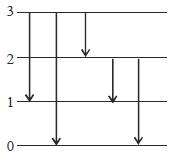Solution: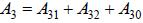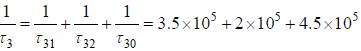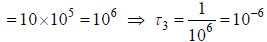QUESTION: 9

The Raman active mode for CO2, molecule is

Solution:

Symmetric streching mode lead to change in Polarisability and hence Raman active

QUESTION: 10

CO2 molecule lias the first tew energy levels uniformly seperatedby approximately 2.5 meV at a tempera tine of300 K. the ratio of the number of molecules in the 4th excited state to the number in 2nd excited state is about

Solution: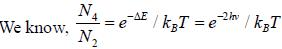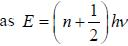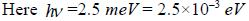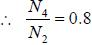QUESTION: 11

The ground state terms for nd10, np4, np3 and nd8 are respectively in L-S coupling are

Solution: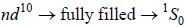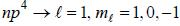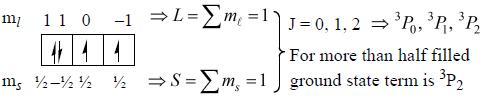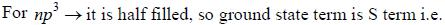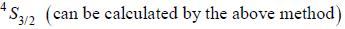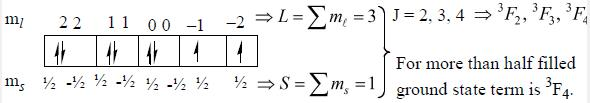QUESTION: 12

If the wavelength of the first line of Lyman series of hydrogen is 1215 Å. the wavelength of the second line of the series is

Solution: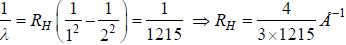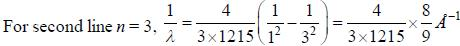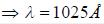QUESTION: 13

The Kβ line of X-ray emitted from an atom with principal quantum number n = 1, 2, 3,   arise from the transition.

Solution:

We Know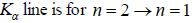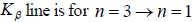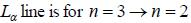QUESTION: 14

If the line taken by an electron to traverse the first orbit in hydrogen atom is T. then the time taken by the electron to traverse the second orbit in lithium atom (Li++) is

Solution: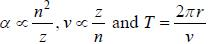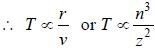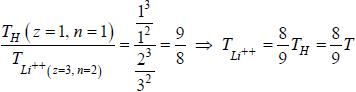QUESTION: 15

If the atom is in the 3D3 state, the angle between it’s orbital and spin angular momentum vectors is

Solution: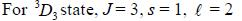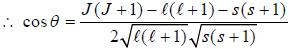On putting all these value, we get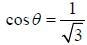QUESTION: 16

Which of the following transition are allowed

Solution:

Selection rule is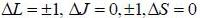QUESTION: 17

Sodium atom ground state configuration is 1s2 2s2P6 3s1. by emitting 1e- Na changes to Na+ having configuration 1s2 2s2 2P6 in the transition the change in J value is

Solution:

Ground state term for sodium is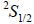while the new state Nahas ground term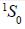∴ Change in J value in this transition =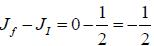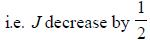QUESTION: 18

According to the electric dipole transition, which is correct

Solution:
*Answer can only contain numeric values
QUESTION: 19

Nuclear spin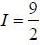for bismuth atom The number of levels in which the term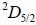of bismuth will split up?

Solution: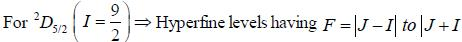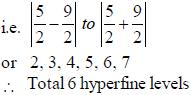QUESTION: 20

For pure rotatioal Raman spectrum of a linear diatomic molecule is recorded using Electromagnetic radiation of frequency v6. The frequency of the two consective stokes lines are

Solution: